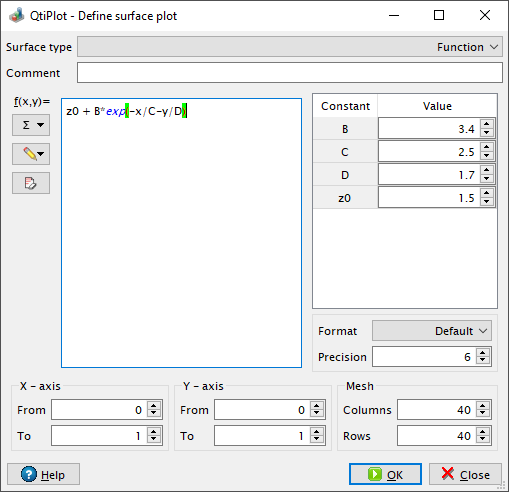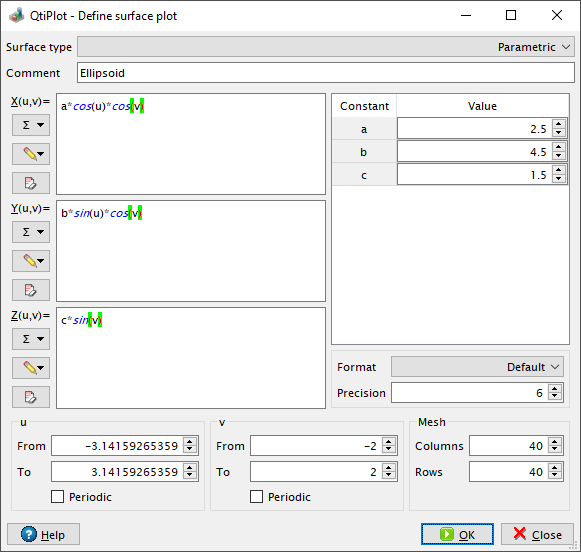# Define surface plot

This dialog is used when you enter the New -> New Function Plot -> New 3D Surface Plot... command. It allows for the creation of a new function of two variables. When the "Function" Surface type is selected the coordinate system will be Cartesian and the function will be of the form: z = f(x,y).

Figure 5-72. The New -> New Function Plot -> New 3D Surface Plot... dialog box.You may also define the X, Y and Z scales and the mesh parameters.

You can create parametric surfaces when the "Parametric" Surface type is selected. The only parameters allowed are latitude and longitude: `u` and `v`. Here, for example, is how you might plot a sphere having a radius equal to one:

Figure 5-73. The New -> New Function Plot -> New 3D Surface Plot... dialog box.As in the case for functions, you can supply the drawing domain for the two angular parameters and you can define the mesh parameters. The more rows/columns you request, the better the resolution of the output image will be. However, this is at the cost of a larger memory consumption and an increased time of computation. A slow CPU and small amounts of memory will be limiting.

Finally, you can provide information to the drawing routines about the rotational symmetry of the parametric surface using the two `Periodic` check box options (one for each parameter).